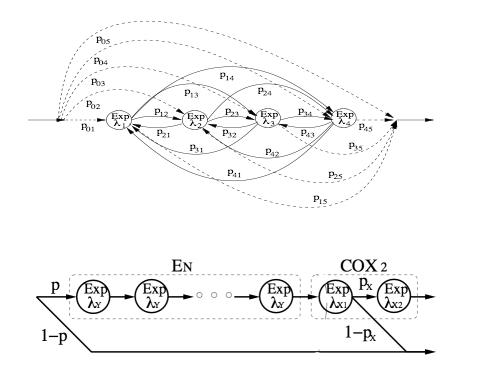## May 15, 2004

### A Closed-Form Solution for Mapping General Distributions to Minimal PH DistributionsI just finished reading A Closed-Form Solution for Mapping General Distributions to Minimal PH Distributions. This was a paper that came from CMU and shows how one can approximate any member of a family of functions. The family of functions is called Phase-Type functions (PH). PH functions are ones that can be represented by a Markov Model that has a structure like that shown at the top of the image to the left. The simpler function comes from a family of functions called the EC family. EC functions are those that can be represented by a Markov Model that has the structure of the model shown at the bottom of the image to the left. An EC function can approximate any PH function such that the first three moments of the EC function match those of the PH function exactly. The greater the variability of the PH function, the less the number of states the EC function requires. Any important conclusion of this paper is that the number of states in the approximating EC model is 1 or 2 more than the minimum needed to optimally represent the PH function as an EC function. Furthermore, all the parameters of the EC function can be computed in closed-form using parameters of the PH function (such as it's first three moments).

The bigger picture for why this is of interest is because of the review of the [rejected] GUIDE AAAI submission. One of the reviewer's comments was that our model wasn't really "continuous" time because it was a DBN and DBN's are a discrete-time model. A DBN cannot handle variables which update at different frequencies without defaulting to updating every variable at the fastest frequency of any variable in the model. A fair criticism I suppose. So this paper is background reading for a sequence of papers about "Continous Time Bayesian Networks" (CTBN) coming out of Stanford and Daphne Koller's group. This is to investigate ways of actually using continuous time directly.

So what the technique in this paper allows one to do is to pick a temporal distribution and to construct a Markov Model with a number of internal states and transitions. The temporal distribution of reaching the sink state of the constructed Markov Model will be [approximately] the same as the initial temporal distribution. So instead of keeping a discretized representation of the original distribution in a conditional probability table (with a discretization at the level of the update frequency of the DBN), there is a Markov Model which has similar temporal properties.

So, here's my issue: It's all well and good that you can create a Markov Model that has the temporal properties that you want, but it's not clear to me how you use it in an inference process in a way that is better than a DBN. A Markov Model requires one to step through the model at a discrete rate also. So the simple idea of replacing a discretized representation of a temporal distribution with an approximate Markov Model representation of the temporal distribution doesn't seem sufficient. In fact, it seems worse because the distribution of the EC Markov Model is less clear than just the explicit discretized temporal distribution.

What I'm assuming must be the case with the CTBN is that the simple structure of the EC Markov Model must allow one to figure out the joint distribution at an arbitrary time in the future using some property of the Markov Model. This isn't possible using a discretized DBN.

Posted by djp3 at 1:20 PM | Comments (0) | TrackBack (0)